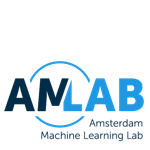## UvA - Machine Learning 1

Welcome to the public page for the course Machine Learning 1. The course is part of the Artificial Intelligence master program at the University of Amsterdam. The course is developed by the Amsterdam Machine Learning Lab and currently taught by dr.ir. Erik Bekkers.

This page presents an overview of the course including links to the lectures (this Youtube channel) and the corresponding annotated slides in pdf. Students enrolled for the course are referred to Canvas for extra materials such as practice exercises, homework assignments, lab assignments (jupyter notebooks) and additional resources.

In this lecture series we follow closely the Pattern Recognition and Machine Learning book by Bishop. Relevant chapters are indicated at the start of each video.### Weekly overview

#### Week 1

• Lecture 1.1 (video, pdf): Introduction to the course, administrative announcements
• Lecture 1.2 (video, pdf): What is Machine Learning?
• Lecture 1.3 (video, pdf): Types of Machine Learning
• Lecture 1.4 (video, pdf): Probability Theory, Bayes Theorem
• Lecture 1.5 (video, pdf): Probability Theory: An Example

• Lecture 2.1 (video, pdf): Expectation, Variance, Covariance
• Lecture 2.2 (video, pdf): Gaussian Distribution
• Lecture 2.3 (video, pdf): Maximum Likelihood Estimation
• Lecture 2.4 (video, pdf): Maximum Likelihood Estimation: An Example
• Lecture 2.5 (video, pdf): Maximum A Posteriori
• Lecture 2.6 (video, pdf): Bayesian Prediction

#### Week 2

• Lecture 3.1 (video, pdf): Linear Regression With Basis Functions
• Lecture 3.2 (video, pdf): Linear Regression via Maximum Likelihood
• Lecture 3.3 (video, pdf): Stochastic Gradient Descent
• Lecture 3.4 (video, pdf): Underfitting and Overfitting
• Lecture 3.5 (video, pdf): Regularized Least Squares

• Lecture 4.1 (video, pdf): Model Selection
• Lecture 4.2 (video, pdf): Bias Variance Decomposition
• Lecture 4.3 (video, pdf): Gaussian Posteriors
• Lecture 4.4 (video, pdf): Sequential Bayesian Learning
• Lecture 4.5 (video, pdf): Bayesian Predictive Distributions

#### Week 3

• Lecture 5.1 (video, pdf): Equivalent Kernel
• Lecture 5.2 (video, pdf): Bayesian Model Comparison
• Lecture 5.3 (video, pdf): Model Evidence Approximation/Empirical Bayes
• Lecture 5.4 (video, pdf): Classification With Decision Regions
• Lecture 5.5 (video, pdf): Decision Theory
• Lecture 5.6 (video, pdf): Probabilistic Generative Models

• Lecture 6.1 (video, pdf): Probabilistic Generative Models: Maximum Likelihood
• Lecture 6.2 (video, pdf): Probabilistic Generative Models: Discrete Data (Naive Bayes)
• Lecture 6.3 (video, pdf): Discriminant Functions
• Lecture 6.4 (video, pdf): Discriminant Functions: Least Squares Regression
• Lecture 6.5 (video, pdf): Discriminant Functions: Perceptron

#### Week 4

• Lecture 7.1 (video, pdf): Classification With Basis Functions
• Lecture 7.2 (video, pdf): Probabilistic Discriminative Models: Logistic Regression
• Lecture 7.3 (video, pdf): Logistic Regression: Stochastic Gradient Descent
• Lecture 7.4 (video, pdf): Logistic Regression: Newton Raphson

• Lecture 8.1 (video, pdf): Neural Networks
• Lecture 8.2 (video, pdf): Neural Networks: Universal Approximation Theorem
• Lecture 8.3 (video, pdf): Neural Networks: Losses
• Lecture 8.4 (video, pdf): Neural Networks: Stochastic Gradient Descent
• Lecture 8.5 (video, pdf): Neural Networks: Backpropagation

#### Week 5

• Lecture 9.1 (video, pdf): Unsupervised Learning - Latent Variable Models
• Lecture 9.2 (video, pdf): K-Means Clustering
• Lecture 9.3 (video, pdf): Lagrange Multipliers
• Lecture 9.4 (video, pdf): Gaussian Mixture Models and The Expectation Maximization Algorithm

• Lecture 10.1 (video, pdf): Principal Component Analysis: Maximum Variance
• Lecture 10.2 (video, pdf): Principal Component Analysis: Minimum Reconstruction Error
• Lecture 10.3 (video, pdf): Probabilistic Principal Component Analysis
• Lecture 10.4 (video, pdf): Non-Linear Principal Component Analysis (Kernel-PCA and Auto-Encoders)

#### Week 6

• Lecture 11.1 (video, pdf): Kernelizing Linear Models
• Lecture 11.2 (video, pdf): The Kernel Trick
• Lecture 11.3 (video, pdf): Support Vector Machines: Maximum Margin Classifiers
• Lecture 11.4 (video, pdf): Inequality Constraint Optimization (The Dual Lagrangian)
• Lecture 11.5 (video, pdf): Support Vector Machines: Kernel SVM
• Lecture 11.6 (video, pdf): Support Vector Machines: Soft Margin Classifiers

• Lecture 12.1 (video, pdf): Some Useful Properties of Gaussians
• Lecture 12.2 (video, pdf): Kernelizing Bayesian Regression
• Lecture 12.3 (video, pdf): Gaussian Processes
• Lecture 12.4 (video, pdf): Gaussian Processes: With An Exponential Kernel
• Lecture 12.5 (video, pdf): Gaussian Processes: Regression

#### Week 7

• Lecture 13.1 (video, pdf): Model Combination Methods (vs Bayesian Model Averaging)
• Lecture 13.2 (video, pdf): Bootstrapping and Feature Bagging
• Lecture 13.3 (video, pdf): Adaboost
• Lecture 13.4 (video, pdf): Decision Trees and Random Forests